# GSEB Class 10 Maths Notes Chapter 12 Areas related to Circles

This GSEB Class 10 Maths Notes Chapter 12 Areas related to Circles covers all the important topics and concepts as mentioned in the chapter.

## Areas related to Circles Class 10 GSEB Notes

Circle:
Set of all those point which is at constant distance from a fixed point.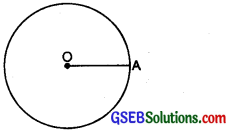Fixed distance from centre of a circle is known as radius of circle. It is denoted by R.

Centre:
Fixed point is known as center (O).

Diameter:
Longest chord passed through centre and whose end point lies on circle is known as diameter of circle.

Circumference of circle:
The circumference of circle bears a constant ratio n with the diameter of the circle i.e. $$\frac{\text { Circumference }}{\text { Diameter }}$$ = π

Diameter or circumference = 2πR or Distance covered in one complete round is called circumference or perimeter.Concentric circle:
Circles having same centre but different radii are known as concentric circle.
Area of Region Enclosed between two concentric circles. If ‘R’ is radius of outer circle and r is radius of smaller (inner) circle.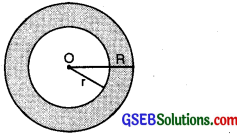Area enclosed between two concentric circles
= πR2 – πr2
= π [R2 – r2] square unit.
Area of circle = πR2

Sector of circle:
Area enclosed between two radii and arc of circle is area of sector APB is minor arc and BQA is major arc. Area enclosed between minor arc APB and two Radii OA and OB is called minor sector.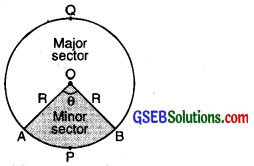Area enclosed between major arc BQA and two radii OA and OB is called major sector formula for finding.
Area of sector = $$\frac{\pi \mathrm{R}^{2} \theta}{360^{\circ}}$$ where θ is sector angle or central angle between two radii

Note:

• If θ = 180° then arc will become semi circle and AOB become diameter.
Area of sector = Area of semicircle
= $$\frac{1}{2}$$ πr2
• If θ is angle of minor sector and (360° – θ) is angle of major sector.

Segment:
Chord AB divides the circular region of the circle in two parts1 ie. region APB and AQB. The AQB is called major segment and the APB region is called minor segment.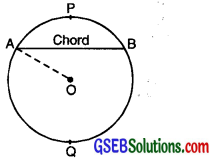Area of minor segment. Area of minor segment APB = Area of the minor sector OAPB – Area of AOAB.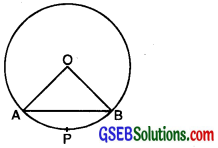= $$\frac{\pi R^{2} \theta}{360}$$ – Area of ∆OAB

Length of Arc.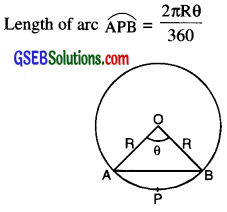Area of square = (side)2
Area of Rectangle = L × B
Area of Parallelogram = Base × Altitude
Area of right angled triangle
= $$\frac{1}{2}$$ Base × Altitude

Area of Equilateral triangle
= $$\frac{\sqrt{3}}{4}$$ (side)2

Perimeter of triangle = sum of three sides of triangle.Areas of Combinations of Plane figures
In our daily life, we saw various interesting designs like flower beds, drain covers, window designs etc. Some times, we want to find the areas of these designs then firstly we divide whole designs to known Plane figures whose areas are previously known by us. Then, calculate the area of each part and by adding these areas, we get the area of required design. We illustrate the process of calculating areas of these types of figures through given problems.